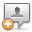KB Home | Advanced Search | News | Ask Question ProSoft Knowledge BaseHome » Categories » Multiple Categories

# Is it possible to configure an AFC flow computer solutions to use the ISO 6976 computation standard?

The short answer is, "No, it cannot."  The reason it cannot is this:

ISO 6976 is the ISO standard for calculating compressibilities and energy content of natural gases.  It is similar to the older (pre-1992) edition of AGA 8 (compressibilities) and the current AGA 5 (energy content; but the AFC calculates energy using Appendix C.3 of AGA 8, and not AGA 5).  ISO 6976 is specifically and explicitly designed to "enable tractable calculations to be made without necessitating machine computation", in other words, using the operator's hand calculator.  Consequently, though the calculations are ostensibly simpler than those of AGA 8, ISO 6976 is less general and less flexible than AGA 8 (for example, only 4 reference temperatures are allowed for calculation of energy content -- 0°C, 15°C, 20°C, and 25°C -- and only 3 of those are allowed for calculation of compressibility -- omitting 25°C -- and an appendix labeled "informative", i.e. not part of the official Standard, adds a 5th temperature base, 60°F, but only for energy calculation).

Here is a detailed evaluation of ISO 6976:

1. Energy (heating value, calorific content):
As stated earlier, the AFC uses the methods and values given in AGA 8 (1992) Appendix C.4 to calculate molar heating value.  This calculation is fundamentally identical to that of ISO-6976, with these caveats:
(a) There is some minor variation between the two Standards in some of the numerical values, specifically the pure-component enthalpies at 25°C.  Such numerical properties of pure components must be determined by careful measurement and are expected to be revised from time to time as newer experimental results are accepted as Standard.  We shall research the latest such values accepted as Standard by API.
(b) ISO recommends selecting the pure-component enthalpies from the tabulated column that corresponds to the user's selected reference temperature, but states that these values were calculated from the 25°C values using one or another method (references given), often involving a polynomial expression of Cp (specific heat at constant pressure) as a function of temperature.  ISO's reason for giving tabulated values instead of computation procedure is to simplify the calculations that the user must perform and avoid the need for machine computation (programmed computer).  On the other hand, AGA 8 lists the coefficients of a second-degree (order 2, quadratic) polynomial for Cp over temperature, and the AFC implements the programmed-computer method.  We do not yet know which, if any, of the ISO calculation references the values in AGA 8 are derived from, but ISO states that there is no significant difference among any of their several referenced methods over the temperature range 0°C to 25°C, which is the range of reference temperature permitted by the AFC.  The advantage of AGA 8's method (and therefore that of the AFC) is that the user can choose any reference temperature between 0°C and 25°C instead of being limited to a choice among four specific values (though these four values cover the vast majority of all choices for metric reference temperature, and an appendix gives the enthalpies for the fifth temperature of 60°F), and, incidentally, the programming of the AFC is actually simplified thereby.
(c) Besides heating value on a molar basis, the AFC also calculates it on both a mass and a volume basis.  All three values are characteristic of the fluid, depending only on component analysis and reference temperature (volume depends also on reference pressure); calculations thereof are identical to those specified by ISO (other than units conversion), except for the real-gas density used for the volume calculation (see 2 next).  Energy accumulations and flow rates are calculated by multiplying the appropriate characteristic value with the increment or flow rate of the primary input (mass or volume) scaled as necessary for units of representation.

2. Density and Compressibility (compression factor):
These values are calculated according to AGA 8 (1992) Detail Characterization Method, and not according to the much simpler method of the ISO Standard (which seems to be very similar to that of pre-1992 AGA 8).  ISO's rationale for using the older method is to "enable tractable calculations to be made without necessitating machine computation".  One would expect therefore that greater accuracy can be obtained by using AGA 8, and this expectation is supported by the fact that the compressibilities tabulated in ISO-6976 are to only 4 digits for the major components of natural gas and to 3 digits for the less common components; the "summation factors" used in the ISO calculation are derived from those values, so the uncertainty in the resulting compressibility will be relatively high regardless of accuracy.  Furthermore, ISO lists values only for three common metric reference temperatures (excluding even 60°F) while AGA 8 permits computation for any reasonable reference temperature, as described in 1(b) above.  The only clear advantage of ISO-6976 over AGA 8 is the greatly expanded list of pure components.

Custom Fields
Attachments (0)Comments